Function Repository Resource:

# ButcherTreeData

Get characteristics of Butcher trees, such as the height, width, order, density and number of labelings

Contributed by: Wolfram Research
 ResourceFunction["ButcherTreeData"][tree] gives an association of characteristics of tree. ResourceFunction["ButcherTreeData"][tree,p] gives the characteristic p of tree. ResourceFunction["ButcherTreeData"][tree,{p1,p2,…}] gives the characteristics pi of tree. ResourceFunction["ButcherTreeData"][{tree1,tree2,…},…] gives the characteristics of treei.

## Details and Options

For multiple trees, ResourceFunction["ButcherTreeData"][{tree1,tree2,}] returns an association of the form <|"prop1"{v11,v12,},"prop2"{v21,v22,},|>, where vij is the value of the property i of the tree j.
Possible characterics of tree include:
 "ButcherHeight" the height of the tree "ButcherWidth" the width of the tree "ButcherOrder" the number of vertices in the tree "ButcherGamma" the density of the tree "ButcherSigma" the order of the symmetry group of the tree
Characterics related to the number of possible ways of labeling tree are:
 "ButcherAlpha" labeling with a totally ordered set of labels "ButcherBeta" labeling with all vertices labeled except for the root vertex "ButcherBetaBar" labeling with an unordered set of labels
ResourceFunction["ButcherTreeData"][tree,"ButcherAlpha"] gives the number of ways of labeling the vertices of tree with a totally ordered set of labels such that if (m,n) is an edge, then m<n.
ResourceFunction["ButcherTreeData"][tree,"ButcherBeta"] gives the number of ways of labeling tree with Butcher order -1 distinct labels such that the root is not labeled, but every other vertex is labeled.
ResourceFunction["ButcherTreeData"][tree,{"ButcherBeta",n}] gives the number of ways of labeling n of the vertices of tree with n distinct labels such that every leaf is labeled and the root is not labeled.
ResourceFunction["ButcherTreeData"][tree,"ButcherBetaBar"] gives the number of ways of labeling tree with Butcher order distinct labels such that every vertex is labeled.
ResourceFunction["ButcherTreeData"][tree,{"ButcherBetaBar",n}] gives the number of ways of labeling n of the vertices of tree with n distinct labels such that every leaf is labeled.
The density returned by ResourceFunction["ButcherTreeData"][tree,"ButcherGamma"] is also the reciprocal of the right-hand side of the order condition imposed by tree.
ResourceFunction["ButcherTreeData"][tree] is equivalent to ResourceFunction["ButcherTreeData"][tree,All] giving an association of the chracteristics of the tree.

## Examples

### Basic Examples (3)

Find the height of a Butcher tree:

 In:=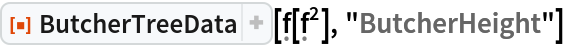Out=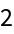Find the width and the height:

 In:=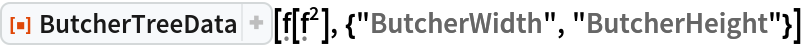Out=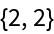Generate trees for any Runge-Kutta method of order 3 using the resource function ButcherTrees:

 In:=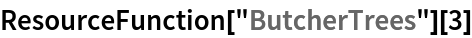Out=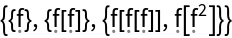Determine their characteristics:

 In:=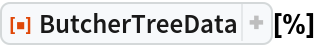Out=Visualize the trees:

 In:=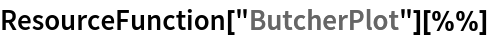Out=### Scope (19)

#### Characteristics of a Tree (3)

Define a Butcher tree and find one of its characteristics (for instance, the order):

 In:=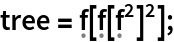In:=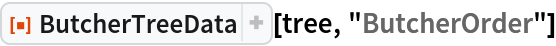Out=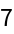Visualize the tree:

 In:=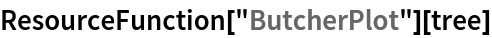Out=Find several characteristics of a tree:

 In:=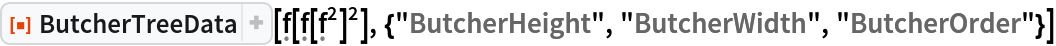Out=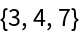Find all the available characteristics of a tree:

 In:=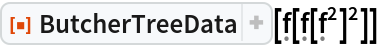Out=In:=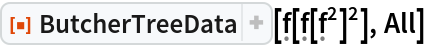Out=#### Characteristics of Several Trees (3)

Generate several trees with the resource function ButcherTrees:

 In:=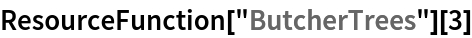Out=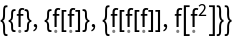Find one characteristic of the list of trees:

 In:=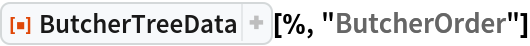Out=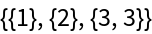With the help of the resource function ButcherTrees, find several characteristics of several trees:

 In:=Out=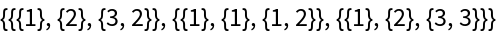Generate several trees with the resource function ButcherTrees and get all of their available characteristics:

 In:=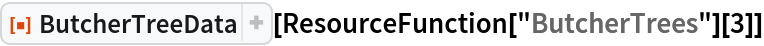Out=In:=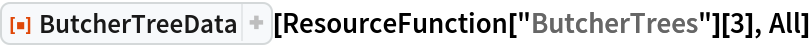Out=#### The Height of Butcher Trees (1)

Find the height of one or more Butcher trees:

 In:=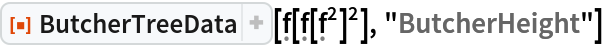Out=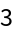In:=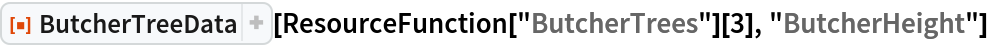Out=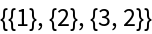#### The Width of Butcher Trees (1)

Find the width of one or more Butcher trees:

 In:=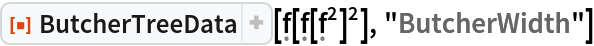Out=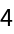In:=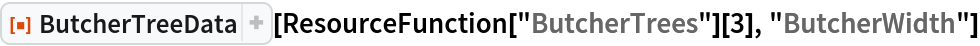Out=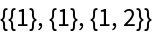#### The Order of Butcher Trees (1)

Count vertices in one or more trees:

 In:=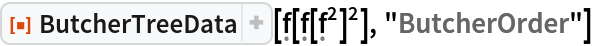Out=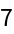In:=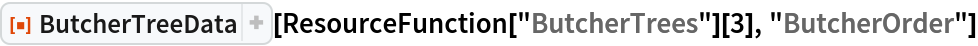Out=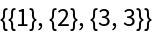#### The Density of Butcher Trees (1)

The density of one or more trees:

 In:=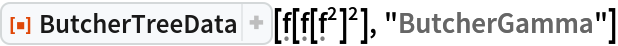Out=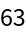In:=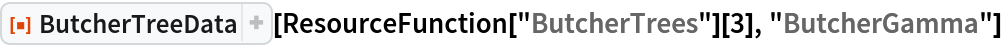Out=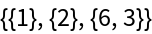#### The Order of the Symmetry Group (1)

Compute the order of the symmetry group of one or more trees:

 In:=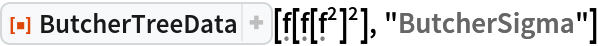Out=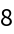In:=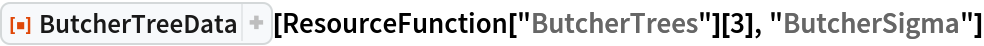Out=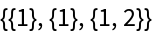#### Labeling with an Ordered Set (1)

The number of ways of labeling the vertices of one or more trees with an ordered set:

 In:=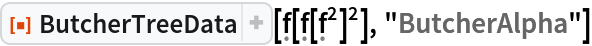Out=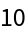In:=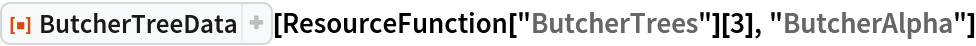Out=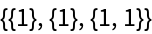#### Labeling Vertices except for the Root Vertex (3)

Compute the number of ways of labeling a tree with Butcher order-1 distinct labels such that all the vertices are labeled except the root:

 In:=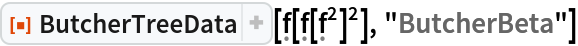Out=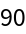Specify the number of labels explicitly:

 In:=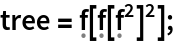In:=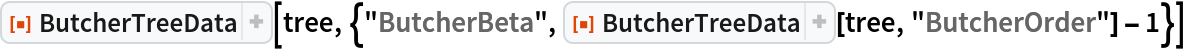Out=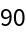Count the number of ways of labeling vertices except the root vertex in several trees:

 In:=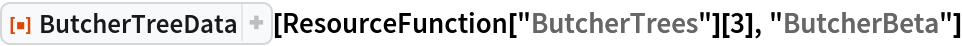Out=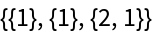#### Labeling with an Unordered Set (4)

Compute the number of ways of labeling a tree with Butcher order distinct labels such that all the vertices are labeled:

 In:=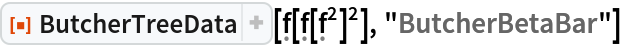Out=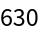Specify the number of labels explicitly:

 In:=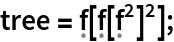In:=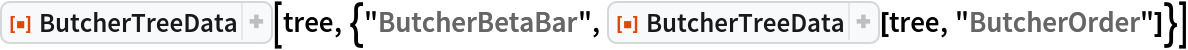Out=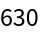Count the number of ways of labeling vertices in several trees:

 In:=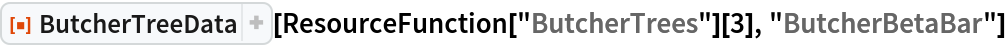Out=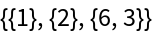Specify the number of labels explicitly:

 In:=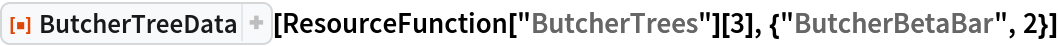Out=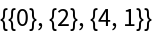## Version History

• 1.0.0 – 04 November 2019